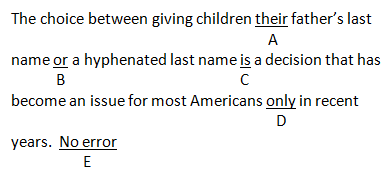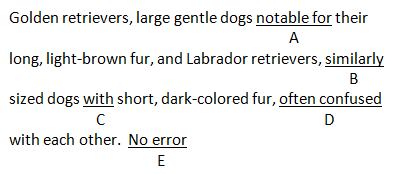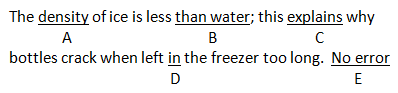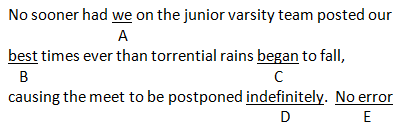# SAT Identifying Sentence Errors: Test 1

NOTE: The new 2016 SAT no longer contains these types of questions. Click here for our updated SAT Writing & Language practice questions.

This free SAT Identifying Sentence Errors practice test includes challenging practice questions and is perfect for your test prep and review. It will test your knowledge of word choice, usage, grammar, and idiom.

Directions: These practice questions will test your ability to recognize grammar and usage errors. Each sentence contains either a single error or no error. None of the sentences contain more than one error. If there is an error, it will be underlined and lettered. Select the underlined portion that must be changed in order to make the sentence correct. If the sentence is already correct, then select choice E.

Congratulations - you have completed . You scored %%SCORE%% out of %%TOTAL%%. Your performance has been rated as %%RATING%%
 Question 1A A B B C C D D E E
Question 1 Explanation:
The correct answer is (B). Although most idioms on the SAT will hinge on preposition usage, some concern verb form (infinitive, gerund) and others involve conjunctions. In this context, “between” requires “and,” not “or”; the error is in (B). (A) uses the correct pronoun for the plural antecedent “children.” (C) agrees with its singular subject “choice.” (D) is the correct idiomatic usage.
 Question 2A A B B C C D D E E
Question 2 Explanation:
The correct answer is (D). A sentence may have multiple nouns and verbs and still be a fragment. This sentence requires a predicate verb, such as “are,” to be complete. (A) and (C) are correct idiomatically, while (B) properly uses an adverb to modify an adjective. The error is in (D).
 Question 3A A B B C C D D E E
Question 3 Explanation:
The correct answer is (B). On the SAT, remember that you may see a plural noun used as an object next to a verb with a singular subject, but don’t be fooled. Here, the plural nouns “defenders” ad “midfielders” are the objects of the verb form “to employ.” The subject of the verb is actually the singular “strategy.” (A) uses the –ing form appropriately. (C) uses the pronoun “she” correctly to refer to the coach. (D) is correct idiomatic usage.
 Question 4A A B B C C D D E E
Question 4 Explanation:
The correct answer is (D). Adjectives can only modify nouns and pronouns; all other parts of speech are modified by adverbs. Here, the adjective “hungry” is used to modify the verb “devoured.” (D) should be the adverb-form “hungrily.” (A) appropriately expresses the contrast between the ideas in the two clauses. (B) uses a consistent verb tense. (C) also uses a consistent tense and agrees with its singular subject, “I.”
 Question 5A A B B C C D D E E
Question 5 Explanation:
The correct answer is (A). Make sure verb tenses in a sentence properly sequence the events. “Will have tasted” indicates an action that will take place in the future, but the sentence discusses events that have already taken place. (B) correctly uses an adverb to modify a verb phrase. (C) is the idiomatically correct preposition in context. (D) uses a consistent verb tense.
 Question 6A A B B C C D D E E
Question 6 Explanation:
The correct answer is (C). When a pair of conjunctions begins with “neither,” it must be completed with “nor.” (A) correctly sets up the contrast between the clauses. (B) agrees with its singular subject “song.” (D) uses the appropriate possessive pronoun with “we” in the first clause.
 Question 7A A B B C C D D E E
Question 7 Explanation:
The correct answer is (B). On the SAT, Comparisons must be made between similar items. Here we are comparing the “density of ice” to “water.” The correct comparison should draw a parallel between the “density of ice” and the “density of water.” The verb of (C) is correct, as is the preposition of (D).
 Question 8
 A A B B C C D D E E
Question 8 Explanation:
The correct answer is (D). Events that occur in the past must have past tense verbs. While the verb in (C) is correctly past tense, the verb “sweeping” is a participle indicating present tense, which is incorrect, and should be the past tense “swept.”
 Question 9A A B B C C D D E E
Question 9 Explanation:
The correct answer is (A). The subject of this sentence is the singular word “menu.” The phrase “for the graduation parties” is simply a prepositional descriptive phrase. The verb should be the singular “has.” (B) and (C) are idiomatically correct, while (D) appropriately modifies the noun “guests.”
 Question 10A A B B C C D D E E
Question 10 Explanation:
The correct answer is (E). Not every Identifying Sentence Errors question on the SAT will contain an error! “We” is the correct subject pronoun for this sentence. “Best” is the superlative form and is correctly used here since more than two “times” are implied (otherwise we’d use the comparative form “better”). For (C), “began” is the correct past tense verb, while (D) is a correctly-used adverb.
Once you are finished, click the button below. Any items you have not completed will be marked incorrect.
There are 10 questions to complete.
 ← List →